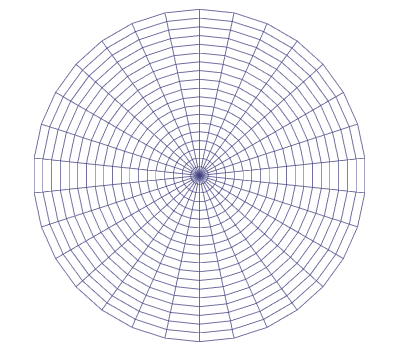# Example PolarGraphGenerator.cs

Shows a simple graph type generating a polar graph.### Version

Tested with version 4.0.10

### See

Writing Graph Generators

`using System.Collections.Generic;using UnityEngine;// Include the Pathfinding namespace to gain access to a lot of useful classesusing Pathfinding;// Required to save the settingsusing Pathfinding.Serialization;using Pathfinding.Util;[JsonOptIn]// Make sure the class is not stripped out when using code stripping (see https://docs.unity3d.com/Manual/ManagedCodeStripping.html)[Pathfinding.Util.Preserve]// Inherit our new graph from a base graph typepublic class PolarGraph : NavGraph { [JsonMember] public int circles = 10; [JsonMember] public int steps = 20; [JsonMember] public Vector3 center = Vector3.zero; [JsonMember] public float scale = 2; // Here we will store all nodes in the graph public PointNode[] nodes; GraphTransform transform; PointNode CreateNode (Vector3 position) { var node = new PointNode(active); // Node positions are stored as Int3. We can convert a Vector3 to an Int3 like this node.position = (Int3)position; return node; } static Vector3 CalculateNodePosition (int circle, float angle, GraphTransform transform) { // Get the direction towards the node from the center var pos = new Vector3(Mathf.Sin(angle), 0, Mathf.Cos(angle)); // Multiply it with the circle number to get the node position in graph space pos *= circle; // Multiply it with the matrix to get the node position in world space pos = transform.Transform(pos); return pos; } protected override IEnumerable<Progress> ScanInternal () { // Create a 2D array which will contain all nodes // This is just a tempoary array to make it easier to reference different nodes PointNode[][] circleNodes = new PointNode[circles][]; // Create a matrix which just moves the nodes to #center // and scales their positions by #scale // The GraphTransform class has various utility methods for working with it transform = new GraphTransform(Matrix4x4.TRS(center, Quaternion.identity, Vector3.one*scale)); // Place the center node in the center circleNodes = new PointNode[] { CreateNode(CalculateNodePosition(0, 0, transform)) }; // The size of the angle (in radians) each step will use float anglesPerStep = (2*Mathf.PI)/steps; for (int circle = 1; circle < circles; circle++) { circleNodes[circle] = new PointNode[steps]; for (int step = 0; step < steps; step++) { // Get the angle to the node relative to the center float angle = step * anglesPerStep; Vector3 pos = CalculateNodePosition(circle, angle, transform); circleNodes[circle][step] = CreateNode(pos); } } // Now all nodes are created, let's create some connections between them! // Iterate through all circles // circle 0 is just the center node so we skip that for now for (int circle = 1; circle < circles; circle++) { for (int step = 0; step < steps; step++) { // Get the current node PointNode node = circleNodes[circle][step]; // The nodes here will always have exactly four connections, like a grid, but polar. // Except for those in the last circle which will only have three connections int numConnections = circle < circles-1 ? 4 : 3; var connections = new Connection[numConnections]; // Get the next clockwise node in the current circle. // The last node in each circle should be linked to the first node // in the circle which is why we use the modulo operator. connections.node = circleNodes[circle][(step+1) % steps]; // Counter clockwise node. Here we check for underflow instead connections.node = circleNodes[circle][(step-1+steps) % steps]; // The node in the previous circle (in towards the center) if (circle > 1) { connections.node = circleNodes[circle-1][step]; } else { // Create a connection to the middle node, special case connections.node = circleNodes[circle-1]; } // Are there any more circles outside this one? if (numConnections == 4) { // The node in the next circle (out from the center) connections.node = circleNodes[circle+1][step]; } for (int q = 0; q < connections.Length; q++) { // Node.position is an Int3, here we get the cost of moving between the two positions connections[q].cost = (uint)(node.position-connections[q].node.position).costMagnitude; } node.connections = connections; } } // The center node is a special case, so we have to deal with it separately PointNode centerNode = circleNodes; centerNode.connections = new Connection[steps]; // Assign all nodes in the first circle as connections to the center node for (int step = 0; step < steps; step++) { centerNode.connections[step] = new Connection( circleNodes[step], // centerNode.position is an Int3, here we get the cost of moving between the two positions (uint)(centerNode.position-circleNodes[step].position).costMagnitude ); } // Store all nodes in the nodes array List<PointNode> allNodes = new List<PointNode>(); for (int i = 0; i < circleNodes.Length; i++) { allNodes.AddRange(circleNodes[i]); } nodes = allNodes.ToArray(); // Set all the nodes to be walkable for (int i = 0; i < nodes.Length; i++) { nodes[i].Walkable = true; } yield break; } public override void GetNodes (System.Action<GraphNode> action) { if (nodes == null) return; for (int i = 0; i < nodes.Length; i++) { // Call the delegate action(nodes[i]); } }}`